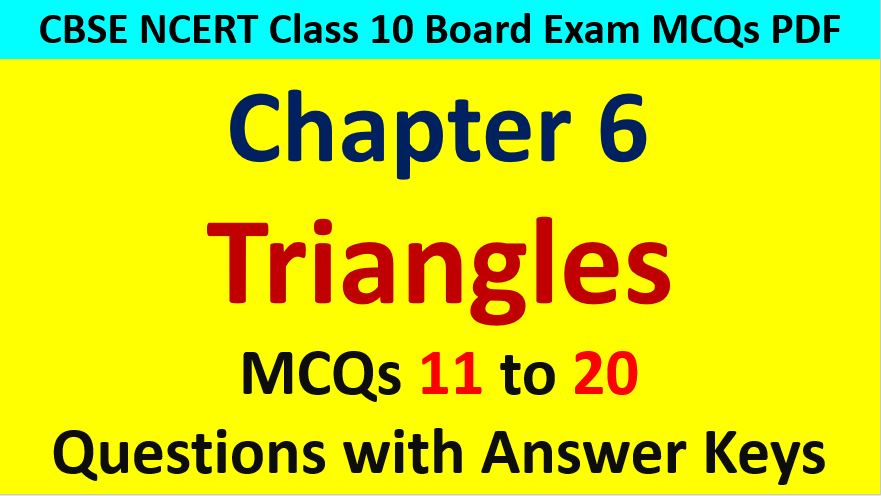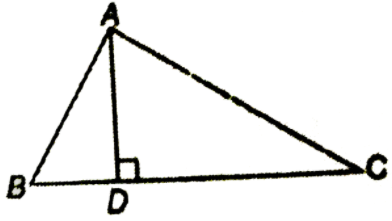Friday, October 22, 2021
Home > CBSE Class 10 > Similar Triangles Class 10 Maths MCQ with Answer Keys Solutions PDF DownloadTriangles MCQ Questions for Class 10 Question No 11:

In figure below, ∠BAC = 90 degree and AD ⟂ BC. Then,Option A : BC.CD = BC2

Option B : AB.BC = BC2

Option C : BC.CD = AD2

Option D : AB.AC = AD2

Option C : BC.CD = AD2

Similar Triangles MCQ Questions for Class 10 Question No 12:

The lengths of the diagonals of a rhombus are 16 cm and 12 cm. Then, the lengths of the side of the rhombus is

Option A : 9 cm

Option B : 10 cm

Option C : 8 cm

Option D : 20 cm

Option B : 10 cm

Triangles MCQ Questions for Class 10 Question No 13:

If ∆ABC ~ ∆EDF and ∆ABC is not similar to ∆DEF, then which of the following is NOT true?

Option A : BC.EF = AC.FD

Option B : AB.EF = AC.DE

Option C : BC.DE = AB.EF

Option D : BC.CE = AB.FD

Option C : BC.DE = AB.EF

Similar Triangles MCQ Questions for Class 10 Question No 14:

If in two triangles ∆ABC and ∆PQR, AB/QR = BC/PR = CA/PQ, then

Option A : ∆PQR ~ ∆CAB

Option B : ∆PQR ~ ∆ABC

Option C : ∆CBA ~ ∆PQR

Option D : ∆BCA ~ ∆PQR

Option A : ∆PQR ~ ∆CAB

Triangles MCQ Questions for Class 10 Question No 15:

In figure below, two lines segments AC and BD intersect each other at the point P such that PA = 6 cm, PB = 3 cm, PC = 2.5 cm, PD = 5 cm, ∠APB = 50 degrees, ∠CDP = 30 degrees. Then, ∠PBA is equal toOption A : 50 degrees

Option B : 30 degrees

Option C : 60 degrees

Option D : 100 degrees

Option D : 100 degrees

Similar Triangles MCQ Questions for Class 10 Question No 16:

If in two triangles ∆DEF and ∆PQR, ∠D = ∠Q and ∠R = ∠E, then which of the following is NOT true?

Option A : EF/PR = DF/PQ

Option B : DE/PQ = PF/RP

Option C : DE/QR = DF/PQ

Option D : EF/RP = DE/QR

Option B : DE/PQ = PF/RP

Triangles MCQ Questions for Class 10 Question No 17:

In triangles ABC and DEF, ∠B = ∠E and ∠F = ∠C and AB = 3DE, then the two triangles are

Option A : congruent but not similar

Option B : similar but not congruent

Option C : neither similar nor congruent

Option D : congruent as well as similar

Option B : similar but not congruent

Similar Triangles MCQ Questions for Class 10 Question No 18:

If ∆ABC ~ ∆PQR with BC/QR = 1/3. Then, ar(∆PRQ)/ar(∆BCA) is equal to

Option A : 9

Option B : 3

Option C : 1/3

Option D : 1/9

Option A : 9

Similar Triangles MCQ Questions for Class 10 Question No 19:

In ∆ABC ~ ∆DEF, ∠A = 30 degrees, ∠C = 50 degrees, AB = 5 cm and AC = 8 cm and DF = 7.5 cm, Then, which of the following is true?

Option A : DE = 12 cm, ∠F = 50 degrees

Option B : DE = 12 cm, ∠F = 100 degrees

Option C : EF = 12 cm, ∠D = 100 degrees

Option D : EF = 12 cm, ∠D = 30 degrees

Option B : DE = 12 cm, ∠F = 100 degrees

Triangles MCQ Questions for Class 10 Question No 20:

If in ∆ABC and ∆DEF, AB/DE = BC/FD, then they will be similar. When,

Option A : ∠B = ∠E

Option B : ∠A = ∠D

Option C : ∠B = ∠D

Option D : ∠A = ∠F